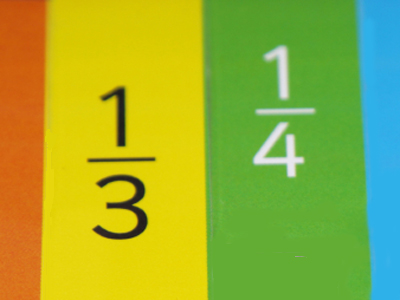The bottom number of a fraction is called the denominator.

# Fractions 4

This Math quiz is called 'Fractions 4' and it has been written by teachers to help you if you are studying the subject at elementary school. Playing educational quizzes is an enjoyable way to learn if you are in the 3rd, 4th or 5th grade - aged 8 to 11.

It costs only \$12.50 per month to play this quiz and over 3,500 others that help you with your school work. You can subscribe on the page at Join Us

Recognizing a fraction when you see one is easy. Fractions represent part of a whole number. But not all fractions are the same type. Proper fractions have top numbers, or numerators, lower than their bottom numbers, or denominators. Improper fractions are those where the numerators are bigger than the denominators. Another way to write improper fractions is as mixed numbers where units are shown next to a proper fraction. For example, 43 is the equivalent of 113.

Take this quiz and test your knowledge of fractions.

1.
Which is an equivalent of 615?
25
37
210
13
6 ÷ 3 = 2 and 15 ÷ 3 = 5 so 25 is the same as 615
2.
What is the numerator?
The top number of a fraction
The bottom number of a fraction
The divider between the two numbers of a fraction
The whole number in a mixed fraction
The numerator tells us how many of the equal parts there are
3.
How do we change 310 to an equivalent fraction?
Divide the numerator by the denominator
Divide the numerator and denominator by the same number
Multiply the numerator by the denominator
Multiply the numerator and denominator by the same number
If we multiply by 10 the equivalent fraction will be 30100
4.
What would 226 be when converted to an improper fraction?
142
156
146
136
146 could also be written as 73
5.
What would 129 be when converted to a mixed number?
339
113
149
219
129 could be written as 139, 113 or 43
6.
Which of these are NOT equivalent to each other?
39 and 13
68 and 34
46 and 812
110 and 25
15 = 210
7.
How do we reduce 520 to an equivalent fraction?
Divide the numerator and denominator by 2
Divide the numerator and denominator by the same number
Multiply the numerator and denominator by 2
Multiply the numerator and denominator by the same number
We have to find the lowest common multiple of 5 and 20 which is 5 so the equivalent will be 14
8.
Which of these statements is FALSE?
One half is 3 times more than one sixth
One third is twice as much as one sixth
One twentieth is half of one tenth
One hundredth is ten times more than one tenth
One tenth is ten times more than one hundredth
9.
How many 11,000 in a whole one?
10
100
1,000
10,000
1,0001,000 = 1, just as 100100 or 33 = 1
10.
What do we call the bottom number of a fraction?
Numerator
Factor
Multiple
Denominator
The denominator tells us the number of parts the whole is divided into
Author:  Amanda Swift